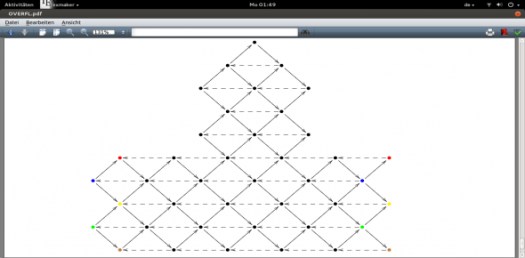# Auslander Reiten Theory Quiz

Approved & Edited by ProProfs Editorial Team
At ProProfs Quizzes, our dedicated in-house team of experts takes pride in their work. With a sharp eye for detail, they meticulously review each quiz. This ensures that every quiz, taken by over 100 million users, meets our standards of accuracy, clarity, and engagement.
| Written by Gregorynaomi
G
Gregorynaomi
Community Contributor
Quizzes Created: 1672 | Total Attempts: 703,062
Questions: 10 | Attempts: 145SettingsAuslander Reiten Theory is a co-work of Maurice Auslander and Idun Reiten in 1975. Their theory studies the representation theory of Artinian rings using the Sequence and quivers techniques. Try this quiz.

• 1.

### What part of Algebra is the Auslander Reiten Theory categorized?

• A.

Linear

• B.

• C.

Complex

• D.

Abstract

D. Abstract
Explanation
Auslander Reiten Theory is categorized under the part of Algebra known as Abstract Algebra. This branch of algebra deals with algebraic structures that are more general and abstract, such as groups, rings, and fields. Auslander Reiten Theory specifically focuses on the representation theory of finite-dimensional algebras, which is a fundamental topic in abstract algebra.

Rate this question:

• 2.

### What other name is given the Auslander Reiten Sequence?

• A.

Almost split sequence

• B.

Mid-range sequence

• C.

Anticlimax sequence

• D.

Degree Sequence

A. Almost split sequence
Explanation
The Auslander Reiten Sequence is also known as the Almost split sequence.

Rate this question:

• 3.

### What does the Auslander Reiten quiver have?

• A.

Variance

• B.

Indiscomposability

• C.

Degree

• D.

Vertex

D. Vertex
Explanation
The Auslander Reiten quiver has vertices. The Auslander Reiten quiver is a directed graph that represents the representation theory of an algebra. Each vertex in the quiver corresponds to an indecomposable module, and the arrows represent the morphisms between the modules. Therefore, the correct answer is "Vertex" as it accurately describes what the Auslander Reiten quiver contains.

Rate this question:

• 4.

### Which of these will warrant adding an arrow in between vertices in an Auslander Reiten quiver?

• A.

When the vertex is negative

• B.

When the sequence is null

• C.

When a morphism is irreducible

• D.

When there is a vector

C. When a morphism is irreducible
Explanation
In an Auslander Reiten quiver, an arrow is added between vertices when there is a morphism that is irreducible. This means that the morphism cannot be factored into smaller nontrivial morphisms. Adding an arrow represents a direct relationship between the two vertices in the quiver, indicating that there is a direct morphism between them that cannot be further decomposed.

Rate this question:

• 5.

### What is the formular of the Auslander Reiten quiver map?

• A.

τ = D Tr ÷∆D

• B.

τ = D Tr

• C.

τ = ∆D Tr

• D.

τ = D.Tr

B. τ = D Tr
Explanation
The formula for the Auslander Reiten quiver map is τ = D Tr. This formula represents a map that takes a representation D of a quiver and maps it to its Auslander Reiten translation Tr(D). The Auslander Reiten translation is a concept in representation theory that describes the relationship between different representations of a quiver. Therefore, the formula τ = D Tr represents the mapping of a representation D to its Auslander Reiten translation Tr(D).

Rate this question:

• 6.

### What is another name for the Auslander Reiten quiver map?

• A.

Sequential Transposition

• B.

Translation

• C.

Translocation

• D.

Coordinate

B. Translation
Explanation
The Auslander Reiten quiver map is commonly referred to as "Translation" in the field of mathematics. This term is used to describe the process of shifting or moving an object or structure along a specified direction or path. In the context of the Auslander Reiten quiver map, it likely refers to the transformation or repositioning of certain elements within the quiver diagram.

Rate this question:

• 7.

### What does D stand for in the Auslander Reiten Theory?

• A.

Dual

• B.

Deviation

• C.

Constant Variable

• D.

Domain

A. Dual
Explanation
In the Auslander Reiten Theory, the letter D stands for "Dual". The theory explores the duality between modules and their duals, which are obtained by reversing the direction of all arrows in the module's quiver. This duality plays a crucial role in understanding the structure and properties of modules and their relationships within a category.

Rate this question:

• 8.

### What does Tr stand for?

• A.

Trigonometric Tripple

• B.

Vector Transpose

• C.

Transpose

• D.

Transpose ratio

C. Transpose
Explanation
Tr stands for transpose, which is a mathematical operation that flips a matrix over its diagonal, reflecting the elements along the main diagonal. This operation is commonly used in linear algebra to manipulate matrices and solve equations. In this context, Tr refers to the transpose of a matrix or a vector.

Rate this question:

• 9.

### Which of these satisfies a sequence to be an Auslander Reiten Sequence?

• A.

• B.

The sequence is not split

• C.

The sequence is in decreasing order

• D.

The sequence is split

B. The sequence is not split
Explanation
An Auslander Reiten sequence is a sequence of modules in a representation of an algebra that satisfies certain properties. One of these properties is that the sequence is not split. This means that there are no non-zero homomorphisms between consecutive modules in the sequence. Therefore, the correct answer is that the sequence is not split.

Rate this question:

• 10.

### Which of these is true for 0→ A → B → C → 0?

• A.

The module B in the almost split sequence is isomorphic to D Tr A

• B.

The module A in the almost split sequence is isomorphic to D Tr C

• C.

The module A in the almost split sequence is interjective to D Tr B

• D.

The module A in the almost split sequence is not isomorphicBack to top